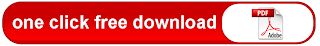# Summary of basic C++-commands / PDF

Summary of basic C++-commands / PDFCompiling

To compile a C++-program, you can use either g++ or c++.
g++ -o executable filename.out sourcefilename.cc
c++ -o executable filename.out sourcefilename.cc
e.g. g++ -o C++sample inout.out C++sample inout.cc
For the following commands you can find at the end of this summary sample programs.

Each command in C++ is followed by “;” . Carriage return has no meaning in C++.

Essential for the writing of clear programs are comments, which are explana-
tions for your program, and which are ignored by the compiler.
/* . . . */ puts the text . . . into comment (more than one line possible)
// . . . puts text . . . for rest of same line into comment

Data Types
Data Type Declaration (Example) Assignment (Example)
integer short i1,i2; i1 = 3;
int i1,i2;
long i1,i2;
unsigned i1,i2;
unsigned long i1,i2;
real float f1,f2; f1 = 3.2; f2 = 2.1E-3;
double f1,f2;
long double f1,f2;
single character char c1,c2; c1 = ’R’
string of characters string s1,s2; s1 = ”Farmer”
logical bool b1,b2; b1 = true; b2 = false

Input And Output
Input/Output With Screen:
To be able to use the following commands you need to write
#include
using namespace std;
at the beginning of your program:
output: cout << ”string of characters”;
cout << variable; << endl;
input: cin >> variable;

Input/Output With Files:
To be able to use the following commands you need to write
#include at the beginning of your program:
output: ofstream outfilevariable ( ”outputfilename”,ios::out);
outfilevariable << . . . will write into file with name outputfilename
input: ifstream infilevariable ( ”inputfilename”,ios::in);
infilevariable >> . . . will read from file with name outputfilename

Arithmetic Calculations
Operations: + − ∗ /
Functions:
To be able to use all of the following functions you need to to write at the
#include
#include
pow(x,y) xy
sin(x)
cos(x)
tan(x)
asin(x) sin−1(x) in range [− /2, /2]
acos(x) cos−1(x) in range [0, ]
atan(x) tan−1(x) in range [− /2, /2]
sinh(x)
cosh(x)
tanh(x)
exp(x) ex
log(x) ln(x)
sqrt(x) √x
fabs(x) |x|
floor(x) largest integer not greater than x; example: floor(5.768) = 5
ceil(x) smallest integer not less than x; example: ceil(5.768) = 6
fmod(x,y) floating-point remainder of x/y with the same sign as x
x % y remainder of x/y, both x and y are integers; example: 7%5=.......Summary of basic C++-commands / PDF

This post first appeared on Free Tutorial 4U, please read the originial post: here

# Share the post

Summary of basic C++-commands / PDF

×